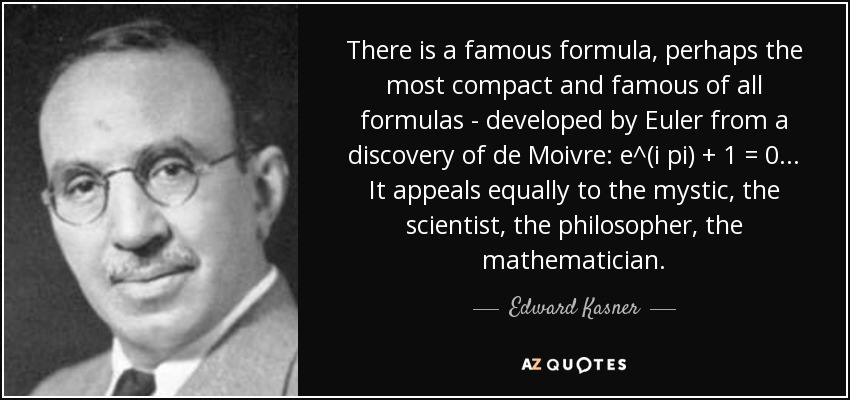Authors:
• There is a famous formula, perhaps the most compact and famous of all formulas - developed by Euler from a discovery of de Moivre: e^(i pi) + 1 = 0... It appeals equally to the mystic, the scientist, the philosopher, the mathematician.

### TopicsEmbed: